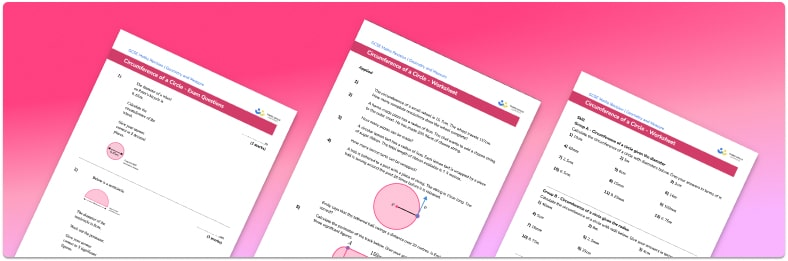# Circumference Of A Circle Worksheet• Section 1 of the circumference of a circle worksheet contains 20+ skills-based circumference of a circle questions, in 3 groups to support differentiation
• Section 2 contains 3 applied circumference of a circle questions with a mix of word problems and deeper problem solving questions
• Section 3 contains 3 foundation and higher level GCSE exam style circumference of a circle questions
• Answer keys and a mark scheme for all circumference of a circle questions are provided
• Questions follow variation theory with plenty of opportunities for students to work independently at their own level
• All questions created by fully qualified expert secondary maths teachers
• Suitable for GCSE maths revision for AQA, OCR and Edexcel exam boards

• This field is for validation purposes and should be left unchanged.

You can unsubscribe at any time (each email we send will contain an easy way to unsubscribe). To find out more about how we use your data, see our privacy policy.

### Circumference of a circle at a glance

The circumference of a circle is the distance around the outside of the circle, similar to the perimeter of a polygon. The circumference of a circle can be found using either the formula C=πd where d is the diameter of the circle or the formula C=2πr where r is the radius of the circle

For example, the diameter of a circle is 10cm. The radius of the circle is therefore 5cm. We can calculate the circumference of the circle using C=π*10 or C=2*π*5. Both give the circumference of this circle as C=31.141592654…cm.

Since both of these formulae use π, which is an irrational number (cannot be written as a fraction), any calculation for the circumference will give a decimal answer. We may be asked to round the answer to the nearest integer, to a given number of decimal places or significant figures or to give an exact answer in terms of π

We usually use metric units in maths and circumference is a measure of length so common units of measurement for the circumference of a circle are mm, cm or m.

Looking forward, students can then progress to additional circles, sectors and arcs worksheets and other geometry worksheets, for example an angles in polygons worksheet or area and circumference of a circle worksheet.For more teaching and learning support on Geometry our GCSE maths lessons provide step by step support for all GCSE maths concepts.

## Do you have KS4 students who need more focused attention to succeed at GCSE?There will be students in your class who require individual attention to help them succeed in their maths GCSEs. In a class of 30, it’s not always easy to provide.

Help your students feel confident with exam-style questions and the strategies they’ll need to answer them correctly with our dedicated GCSE maths revision programme.

Lessons are selected to provide support where each student needs it most, and specially-trained GCSE maths tutors adapt the pitch and pace of each lesson. This ensures a personalised revision programme that raises grades and boosts confidence.

Find out more